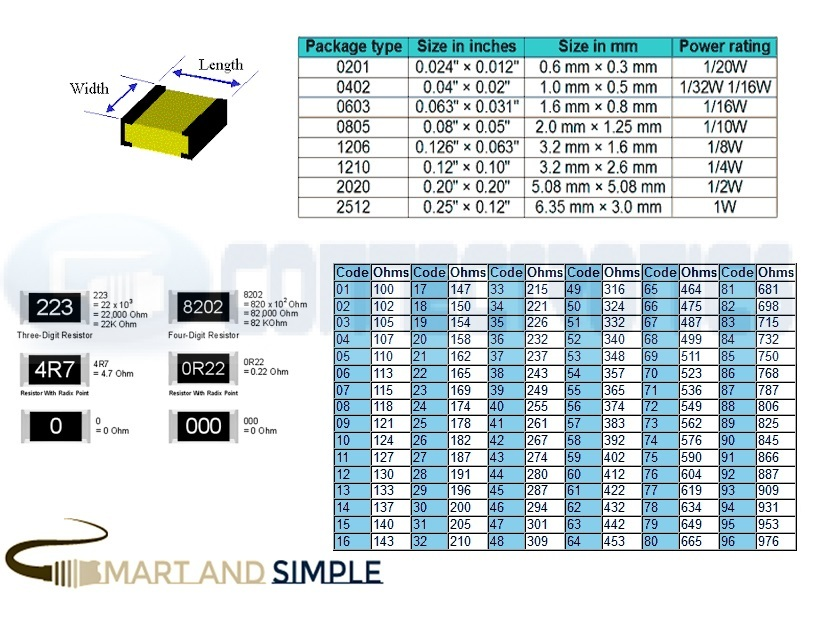# Type of SMD Resistor-electronic componentsmeasure dimension to check Case

Marking 2 digit= refer code

Marking 3 digit= example 223 = 22 x 10^3 = 22,000 ohm = 22k ohm

Marking 3 digit (WITH R) = example 4R7 = 4.7 ohm

Kong
Hello
_______________________________________________________________________________________

••